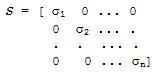# Singular Value Decomposition (SVD)

 Let's suppose that a matrix A is singular. Then, let A be a real m x n matrix of rank r, with m greater than or equal to n. The Singular Value Decomposition (svd) of A is A = U S V' (the apostrophe after a matrix or vector means its transpose) where U is an orthogonal m x n matrix, S is an r x r diagonal matrix, and V is an n x n square orthogonal matrix.

Since U and V are orthogonal, then

UU' = I and VV' = I

That is, the transpose of each matrix is equal to its inverse. The elements along the diagonal of S, labelled, are called the singular values of A. There are r such singular values and they satisfyIf the matrix A is square, then we can use the singular value decomposition to find the inverse, which is is

A-1 = (USV')-1 = (V')-1S-1U-1 = VS-1U'

since (AB)-1 = B-1A-1UU' = I, and VV' = I

If A is a square matrix thenAnd so,If an SVD of a matrix A can be calculated, so can be its inverse. Therefore, we can find a solution to a system

Ax = b  => x = A-1b = VS-1U'b

that would otherwise be usolvable.

Example:

Let's find with Matlab the singular value decomposition of

A = [ 0   -1
-2    1
1    0]

We simply type:

[U,S,V] = svd(A)

and the above operation produces a diagonal matrix S, of the same dimension as A and with nonnegative diagonal elements in decreasing order, and unitary matrices U and V so that A = U*S*V'.

The Matlab answer is:

U =
-0.1826   -0.8944    0.4082
0.9129    0.0000    0.4082
-0.3651    0.4472    0.8165

S =
2.4495         0
0    1.0000
0         0

V =
-0.8944    0.4472
0.4472    0.8944

>>

We can confirm the values of UU', VV' and USV, by executing these instructions in Matlab

U*U'
V*V'
U*S*V

The confirming responses are:

ans =
1.0000   -0.0000   -0.0000
-0.0000    1.0000   -0.0000
-0.0000   -0.0000    1.0000

ans =
1     0
0     1

ans =
-0.0000   -1.0000
-2.0000    1.0000
1.0000    0.0000

From 'Singular Value Decomposition' to home

 Top LU Factorization Matrix decomposition